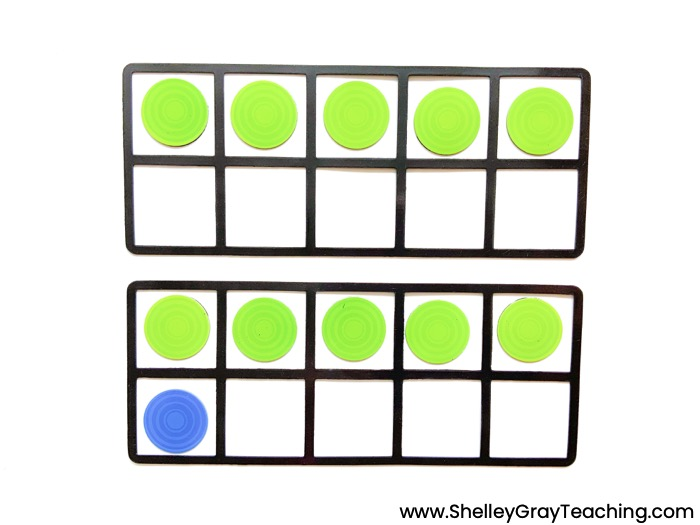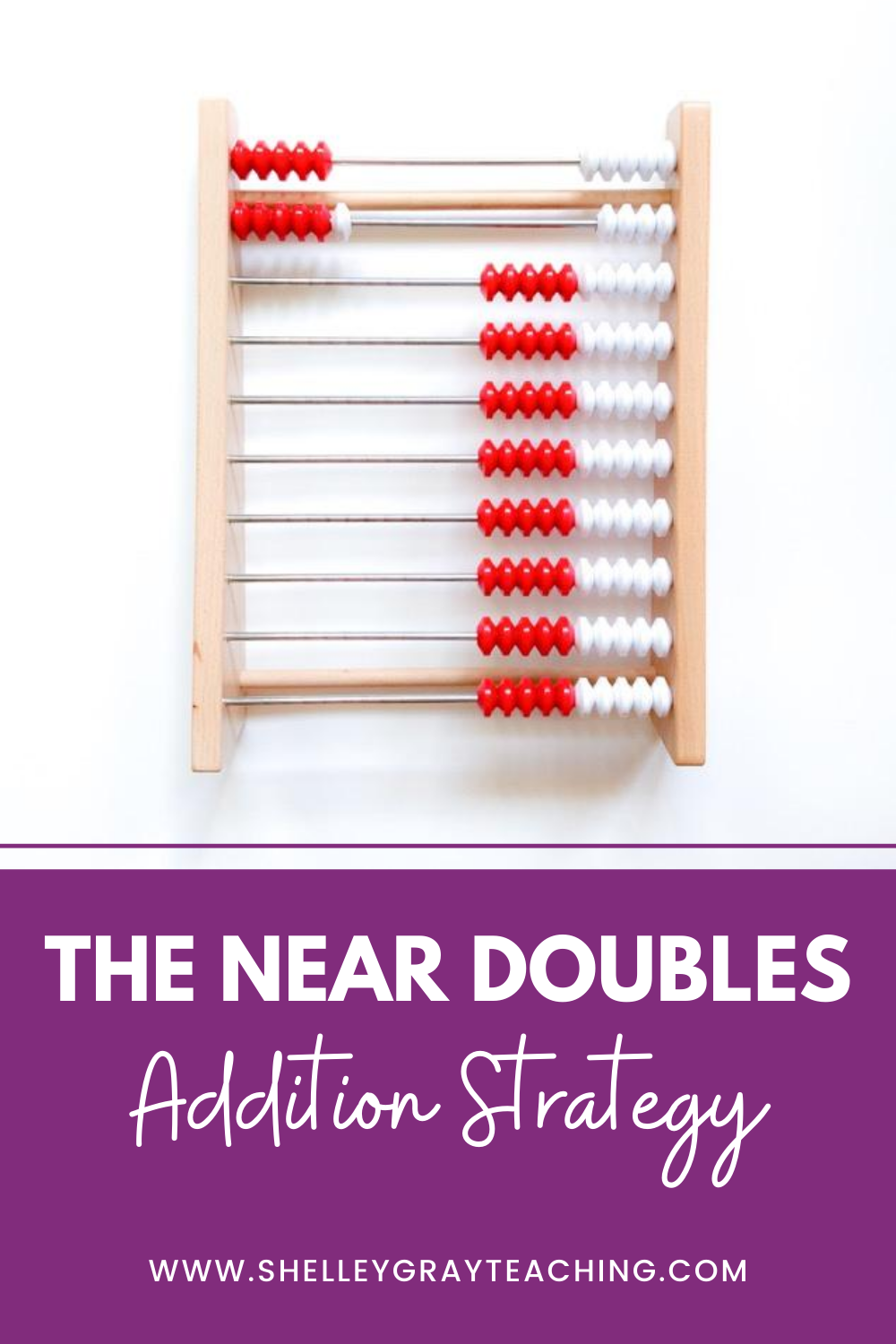# The Near Doubles Addition Strategy### WHAT IS A NEAR DOUBLE?

A near double is a math fact that is close to a doubles fact. For example, 6+7 is considered a near double because it is close to the doubles fact 6+6.

Near doubles could be doubles plus one facts, doubles plus two facts, or doubles minus one facts.

Let’s take a look at the doubles fact 5+5. 5+6 would be the double plus one (5+5 plus one more). 5+7 would be the double plus two (5+5 plus two more). 5+4 would be the double minus one (5+5 subtract one).

### EXPLORATION VS MEMORIZATION

The near doubles strategy teaches us that we can make connections and form relationships between facts. However, we must be careful that we are not teaching this in a procedural way where our students “memorize” this strategy.

If you hear your students saying things like, “I can’t remember the strategy to use when the numbers are one apart!” or “I know I’m supposed to use the doubles plus one strategy for this problem, but I can’t remember how!” then they are not understanding this strategy in a conceptual way. Instead, they are trying to memorize it and apply it.

Ideally, we want to give our students the opportunity to explore this strategy and construct their own understanding. The best way to do this is through lots (and lots!) of time with manipulatives.

### TEACHING THE NEAR DOUBLES IN A CONCEPTUAL WAY

Take a look at this ten frame. The colors make it very easy to see 5+6 as 5+5 and then one more.Here’s another example where we can see 3+5 as 3+3 and then two more.We could also model near doubles on a rekenrek. Here’s a representation of 5+6, which we can clearly see as 5+5 and then one more.### BIG GOAL

When it comes to math fact fluency, our big goal is real understanding and flexibility. We want our students to see pictures in their minds rather than only digits.

To help our students see these pictures in their minds, we need to give them time to work with manipulatives and see the math in different ways. I talk about this in-depth in my post on the Concrete Representational Abstract Model.

Here’s a video with ideas for teaching this strategy in a conceptual way.

Let’s get away from math fact memorization and move towards math fact understanding!